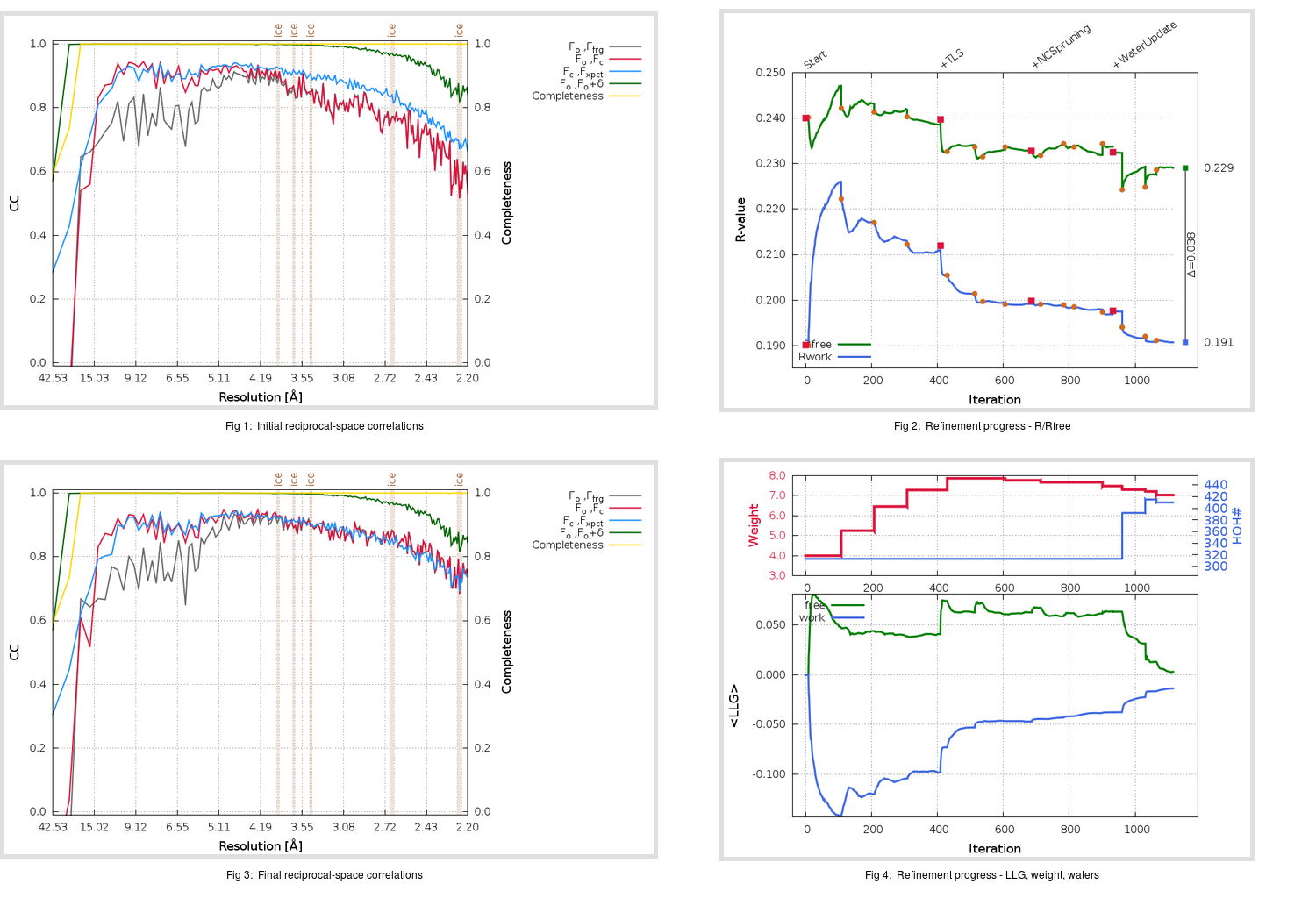Content:

```    Diffraction limits & principal axes of ellipsoid fitted to diffraction cut-off surface:
2.104         1.0000   0.0000   0.0000       a*
2.488         0.0000   1.0000   0.0000       b*
2.139         0.0000   0.0000   1.0000       c*
```

## Deposited

` `
 Date deposited Date data collection Resolution R, Rfree 20200215 20200201 2.20 0.1862 0.2473

Molprobity (CCP4 7.0 version) summary:

```Ramachandran outliers =   0.00 %
favored =  95.50 %
Rotamer outliers      =   4.24 %
C-beta deviations     =     1
Clashscore            =   4.82
RMS(bonds)            =   0.0157
RMS(angles)           =   2.03
MolProbity score      =   2.04
Resolution            =   2.20
R-work                =   0.1862
R-free                =   0.2473
```

```Number of waters      =   313

<B> (all atoms) =   44.31 ( sd =   15.93 ) for       5072 non-hydrogen atoms
<B>   (protein) =   44.25 ( sd =   16.36 ) for       4673 non-hydrogen atoms
<B>     (water) =   43.79 ( sd =    8.89 ) for        313 non-hydrogen atoms
<B>    (others) =   49.36 ( sd =   10.00 ) for         86 non-hydrogen atoms

B min/max       (all non-hydrogen atoms) =   20.79 /  132.19
B min/max   (protein non-hydrogen atoms) =   20.79 /  132.19
B min/max     (water non-hydrogen atoms) =   25.70 /   75.22
B min/max     (other non-hydrogen atoms) =   34.93 /   77.63
```

## BUSTER (re-)refinement

` `

Molprobity (CCP4 7.0 version) summary:

```Ramachandran outliers =   0.00 %
favored =  98.17 %
Rotamer outliers      =   2.50 %
C-beta deviations     =     0
Clashscore            =   2.25
RMS(bonds)            =   0.0120
RMS(angles)           =   1.59
MolProbity score      =   1.31
Resolution            =   2.20
R-work                =   0.1908
R-free                =   0.2292
```

```Number of waters      =   410

<B> (all atoms) =   45.67 ( sd =   14.43 ) for       5169 non-hydrogen atoms
<B>   (protein) =   45.19 ( sd =   14.66 ) for       4673 non-hydrogen atoms
<B>     (water) =   51.19 ( sd =   11.81 ) for        410 non-hydrogen atoms
<B>    (others) =   44.99 ( sd =    3.95 ) for         86 non-hydrogen atoms

B min/max       (all non-hydrogen atoms) =   22.02 /  100.97
B min/max   (protein non-hydrogen atoms) =   23.30 /  100.97
B min/max     (water non-hydrogen atoms) =   22.02 /   90.89
B min/max     (other non-hydrogen atoms) =   39.79 /   53.62
```

Refinement progression:Results:

` `
 File Remark 6Y2G_aB_refine.01_04_refine.pdb.gz exact refinement commands are in header 6Y2G_aB_refine.01_04_refine.mtz.gz including original deposited data and several re-refinement map coefficients 6Y2G_aB_refine.01_04_BUSTER_model.cif.gz including any non-standard compound restraints 6Y2G_aB_refine.01_04_BUSTER_refln.cif.gz